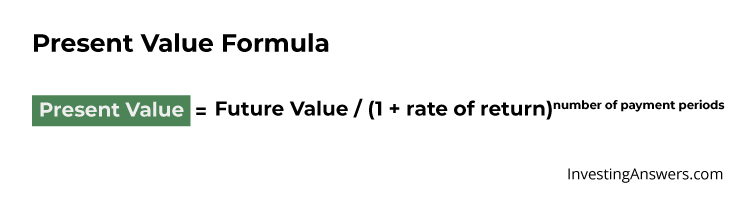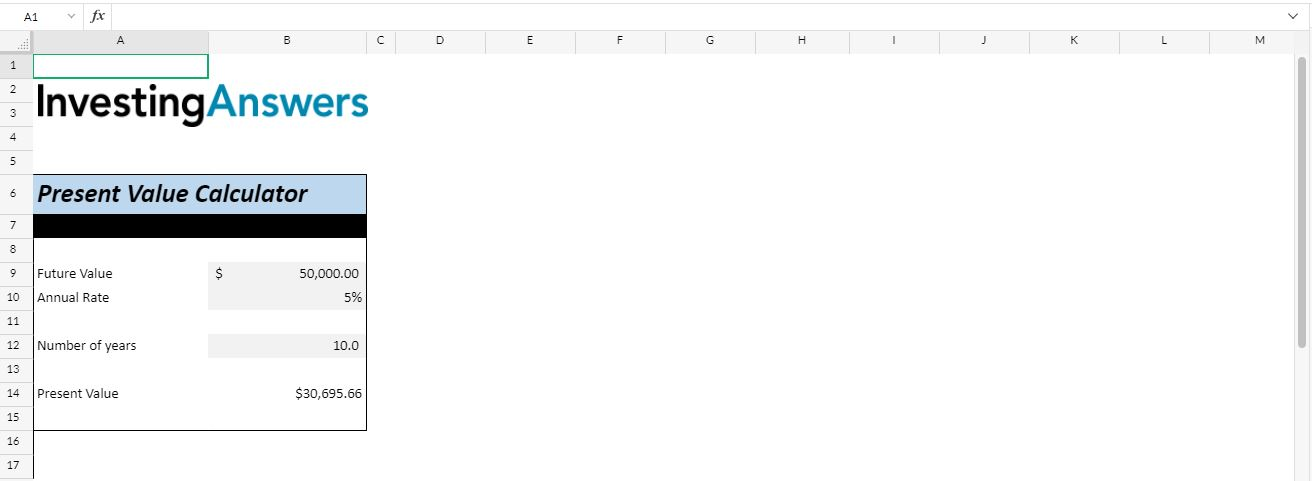# Present Value (PV)

Updated June 22, 2021

## What Is Present Value?

Present value (PV) measures the current value of an amount of money – or a stream of cash flows – that is expected in the future. This value will differ from the cash flows’ nominal value, since time itself affects value. Time represents distance from money, and distance creates risk, which offsets value. In investing, risk is compensated by interest or returns to investors.
Present value is the comparable value today of cash sometime in the future.

## Why Present Value Is Important

Present value is important because it allows investors to compare values over time. PV can help investors assess future financial benefits of current assets or liabilities. Used in areas like financial modeling, stock valuation, and bond pricing, based on its future returns, investors can calculate present value.

For instance, if an investor needs to compare two types of investments, she can assess which one offers the best returns by comparing their present values.

## How to Calculate Present Value

The PV formula discounts the future value of an asset to what it would be worth today.  Calculating present value involves looking at an implied annual rate of return (whether that’s inflation or expected interest earned from an investment). This part of the formula is also referred to as the “discount rate,” or the rate at which time affects value, where the present value with discount rate formula would yield the forgone rate of return should you accept a sum of money in the future compared to today.

Other elements in the present value formula include looking at the number of payment periods and the future value (aka the projected amount of money in the future).

## Present Value Formula

The present value formula is as follows:### Present Value Formula Example

You expect to receive \$50,000 ten years from now, assuming an annual rate of 5%, you can find the value of that sum today.

Use the formula as follows:
PV = \$50,000 /(1 + 0.05)10

= \$30,695.66

This means that the present value of your investment is \$30,695.66.

How to Calculate PV in Excel

The most accurate (and easiest) method to calculate present value is with a financial calculator or Microsoft Excel. In fact, Excel has a built-in function to help you calculate PV.

Let’s use the example above to learn how to calculate PV in Excel. Here’s what you do:

• Write the word “Year” in the first cell (A1), then write years 0 to 10 in cells B1 to K1.

• Write the word “Interest Rate” in cell A2, then enter 5% for cells C2 to L2

• Write the word “Future Value” in cell A3 and write \$50,000 in cell L3

Write the word “Present Value” in cell A4 and then enter in the formula “=-PV(L2, K1, 0, L3)” in cell LDoing so should get you \$30,695.66

Present Value Example

Let’s say you’ve invested money in a company a few years ago. Senior leaders of the company want to pay you back \$120,000 in a year, or you can get the money now but it’ll be \$100,000.  If you had the \$100,000 now, you could  invest it at a 5% rate of return for the next 12 months. To determine the best option, you can use the present value formula:

PV = \$120,000 / (1+0.05)1

PV =  \$114,285.71

What this means is that \$120,000 one year from now is worth \$114,285.71 today, so you should not accept the offer of \$100,000, as it is less than the PV of your investment. In other words, you would have to be able to expect a return greater than 5% to take \$100,000 today in lieu of \$120,000 one year from now.

## The Pros and Cons of Present Value

Although calculating present value is important in helping to determine the potential value of an investment, there are some downfalls. Here are some advantages and disadvantages of present value.

Using present value is a relatively quick way to assess the value of an investment today. Using a calculator or Excel, investors can get a quick overview of whether investing money today on a specific asset is worth it, assuming a consistent rate of return.

In other words, present value is an effective way to compare  investment decisions, by evaluating future sums of money in the present.

Calculating PV assumes that you can earn an expected rate of return over a predetermined time period. Therefore, present value may be misleading. Say that you decide to invest in a company and the rate of return ends up changing each year due to industry volatility. In this case, it’s not as easy to calculate present value, since you may not be able to predict a realistic rate of return.

Remember: A return on your investment isn’t guaranteed and inflation can chip away at an investment's rate of return as well.

## Present Value vs. Future Value

Whereas present value calculates what a future sum of money is worth today, future value looks at the value of a current asset at a predetermined date in the future based on an assumed rate of return. The future value formula also assumes there’s a consistent rate of return (in addition to a single amount invested only at the beginning).

Future value allows investors to predict the value of  various investments in the future, whereas present value looks at how much you need today to earn a certain amount of money in the future.

## Present Value vs. Compounding

Compounding can have an effect on the present value because it affects the discount rate. When compounding, the money invested earns interest on future interest, helping to increase its future value. What this means is that the more frequently the rate of return is compounded, the less the present value of a future sum needs to be to achieve a given future value, because compounding effectively increases the rate of return. Likewise, the greater the rate of compounding, the greater the future value of a present sum will be. With infrequent compounding, investors will need more money today to earn an expected future value.

All of our content is verified for accuracy by Rachel Siegel, CFA and our team of certified financial experts. We pride ourselves on quality, research, and transparency, and we value your feedback. Below you'll find answers to some of the most common reader questions about Present Value (PV).

### Can You Increase Present Value?

Yes, you can increase present value by decreasing the discount rate. In other words, you can increase present value by decreasing the interest rate in cash flow analysis.

Rachel Siegel, CFA - 141Rachel Siegel, CFACFA Charterholder

Chartered Financial Analyst

Rachel Siegel, CFA is one of the nation's leading experts at ensuring the accuracy of financial and economic text.  Her prestigious background includes over 10 years creating professional financial certification exams and another 20 years of college-level teaching.